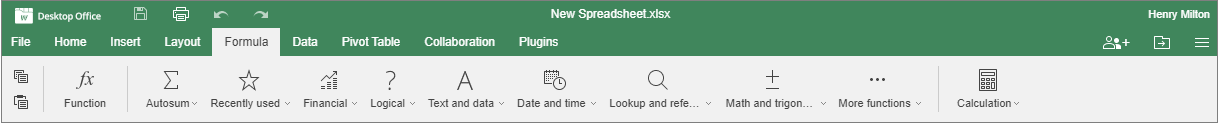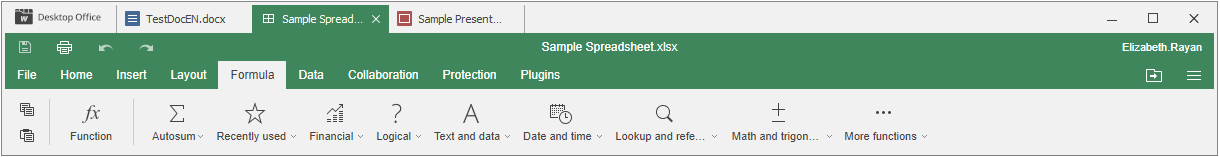### Formula tab

The Formula tab allows working easily with all functions.Figure 11. The corresponding window of the Online Spreadsheet Editor.The corresponding window of the Desktop Spreadsheet Editor.

Using this tab, you can:

• insert functions using the Insert Function dialog window,

• quickly access Autosum formulas,

• access 10 recently used formulas,

• work with formulas classified into categories,

• work with named ranges,

• use the Calculation options: calculate the entire workbook, or the current worksheet only.